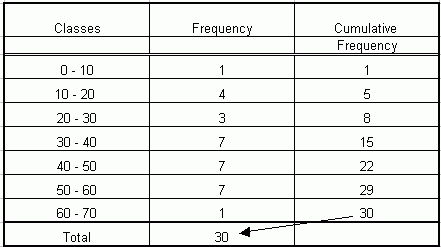Email us to get an instant 20% discount on highly effective K-12 Math & English kwizNET Programs!

#### Online Quiz (WorksheetABCD)

Questions Per Quiz = 2 4 6 8 10

### Middle/High School Algebra, Geometry, and Statistics (AGS)9.7 Less Than Cumulative Frequency

 Cumulative Frequency There are two types of cumulative frequency Less than type cumulative frequency Greater than type cumulative frequency Less than type cumulative frequency The total frequencies of a particular class and all classes prior to that particular class is called the Less than type cumulative frequency of that class or simply the Cumulative frequency of that class. Example The marks of 30 students of a class, obtained in a test out of 75, are given below: 42, 21, 50, 37, 42, 37, 38, 42, 49, 52, 38, 53, 57, 47, 29, 59, 61, 33, 17, 17, 39, 44, 42, 39, 14, 7, 27, 19, 54, 51. A frequency table and a cumulative frequency table with equal class interval is formed.Directions: Answer the questions based on the table above. Also write at least one example of your own.
 Q 1: Which two class intervals have the same minimum frequency ?IIst and VIth Ist and Vth Ist and VIIth Ist and VIth Q 2: What is the lower limit of the sixth class interval?70304050 Q 3: The class mark of the seventh class interval is90655575 Q 4: The cumulative frequency up to the fourth class interval is 51158 Question 5: This question is available to subscribers only! Question 6: This question is available to subscribers only!